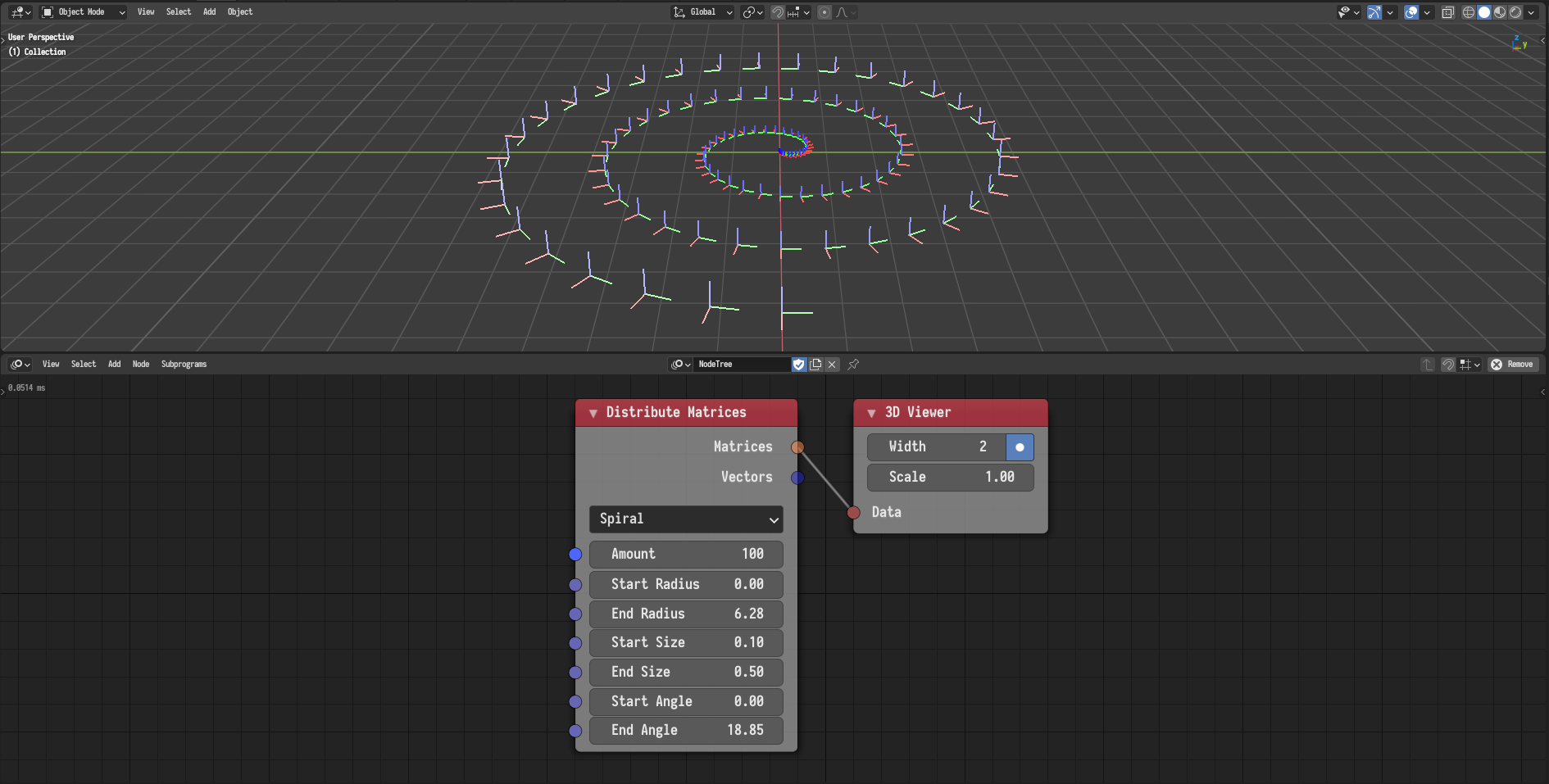# Matrix

## Offset Matrix

The offset transformation can now be defined using an action and can be evaluated either at a certain frame or a range of frames defined by a falloff. The following example illustrates the evaluation of an F-Curves based action at a linear range of frames.

## Distribute Matrices

A new mode for distributing matrices on a spiral was added.A new option for centring grid along some or all of the axis was added.

Finally, the node now returns vector versions of the matrices representing their locations.

## Axis Rotation Matrix

The Axis Rotation Matrix node was added. The node compose a rotation matrix of a rotation around a certain axis.

## Compose Matrix

A new Compose Matrix node was added. The node allows the user to construct a matrix or a list of matrices from locations, rotations, and scales. This node replaces the Translation Matrix, Scale Matrix, and Rotation Matrix nodes.

## Translation Matrix

This node was removed. Replaced by the Compose Matrix node.

## Scale Matrix

This node was removed. Replaced by the Compose Matrix node.

## Rotation Matrix

This node was removed. Replaced by the Compose Matrix node.